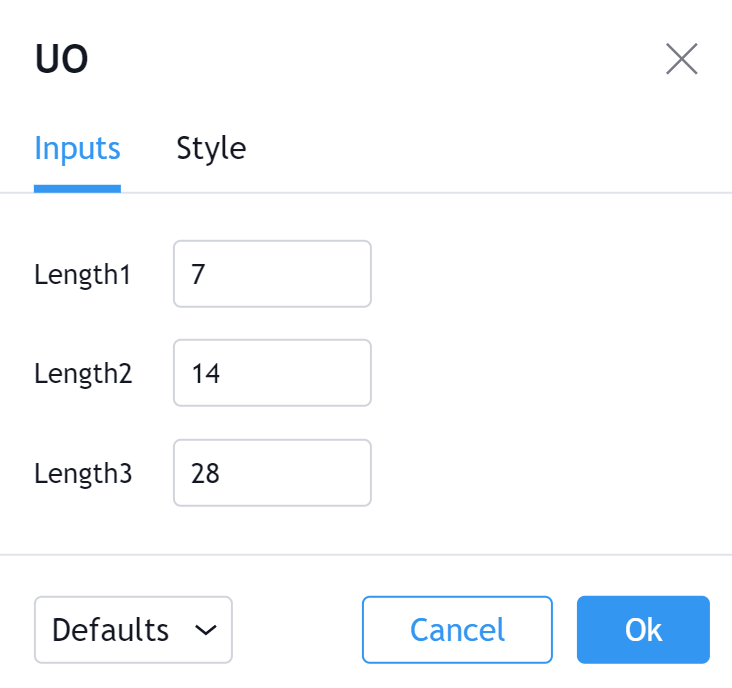# 终极震荡指标(Ultimate Oscillator, UO)

Larry Williams于1976年开发了终极震荡指标(UO)。该指标于1985年在 Stocks & Commodities 杂志上发表。

1. 在计算终极震荡指标之前，需要定义两个变量；买入压力和真实范围。

```Buying Pressure (BP) = Close - Minimum (Lowest between Current Low or Previous Close)
True Range (TR) = Maximum (Highest between Current High or Previous Close) - Minimum (Lowest between Current Low or Previous Close)```

2. 然后，终极震荡指标在三个时间段内使用这些数字：

```Average7 = (7 Period BP Sum) / (7 Period TR Sum)
Average14 = (14 Period BP Sum) / (14 Period TR Sum)
Average28 = (28 Period BP Sum) / (28 Period TR Sum)```

3. 最后，进行终极震荡指标的计算：

`UO = 100 x [(4 x Average7)+(2 x Average14)+Average28]/(4+2+1)`

1. 看涨背离形式意味着价格形成较低的低点，而UO则形成较高的低点。
2. 背离的低点应低于30。
3. UO突破背离高点。

1. 看跌背离形成意味着价格形成较高的高点，而UO形成较低的高点。
2. 背离的高点应高于70。
3. UO低于背离的低点。Length1

Length2

Length3